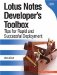# Operators

As with other programming languages, LotusScript employs a number of operators. These operators are used in mathematical equations and to compare data expressions. Some of the more common operators include the following.

Operator

Symbol

Description

+

Used to sum numbers.

Subtract

-

Used to subtract numbers.

Multiplication

*

Used to multiply numbers.

Division

/

Used to divide numbers.

Concatenate

+

Used to merge string values together.

Concatenate

&

Used to merge values together. All values are automatically converted to string and then merged.

Line Continuation

_

The underscore character indicates that a statement continues, or spans, to the next line.

Equal

=

Used to compare two values. For example, if today equals "Tuesday" then perform an action.

Assignment

=

Used to assign a value to a variable. For example, Operator set a text string to a specific valuesuch as "Your document has been saved."

Less Than

<

Used to compare two values.

Greater Than

>

Used to compare two values.

Not Equal

<>

Used to compare two values.

Not Equal

><

Used to compare two values.

Note

In LotusScript, the equal sign = is used as the assignment operator. This differs from the Formula Language syntax, which uses the colon and equal sign := to assign values to variables.Lotus Notes Developers Toolbox: Tips for Rapid and Successful Deployment
ISBN: 0132214482
EAN: 2147483647
Year: N/A
Pages: 293
Authors: Mark Elliott